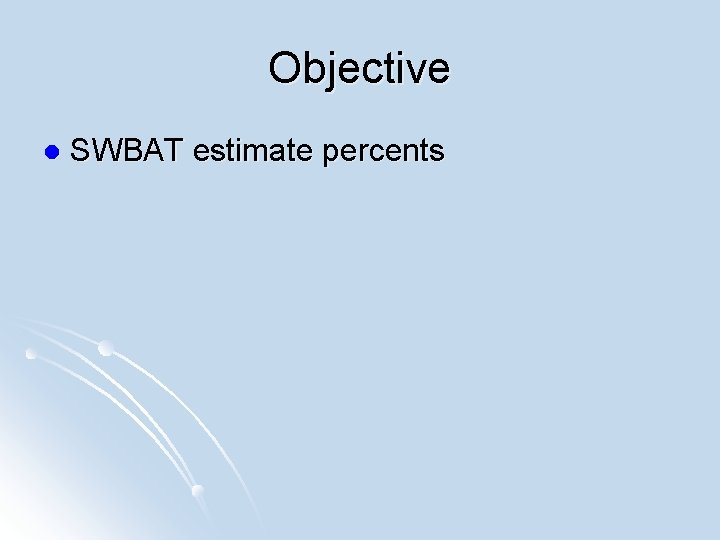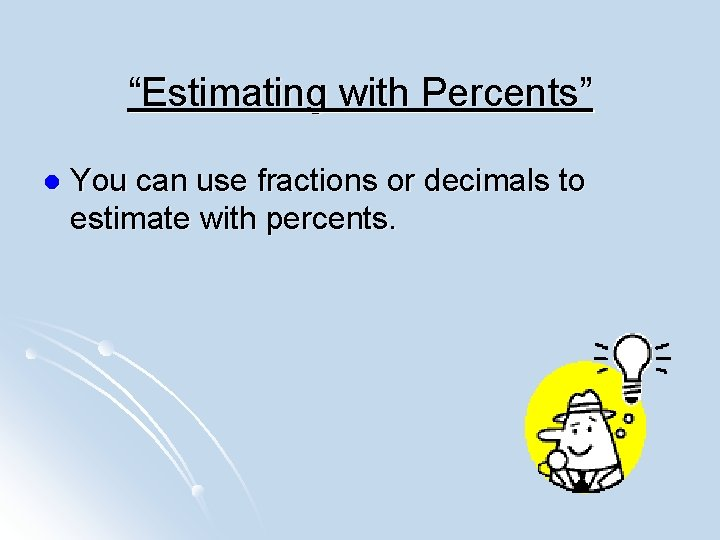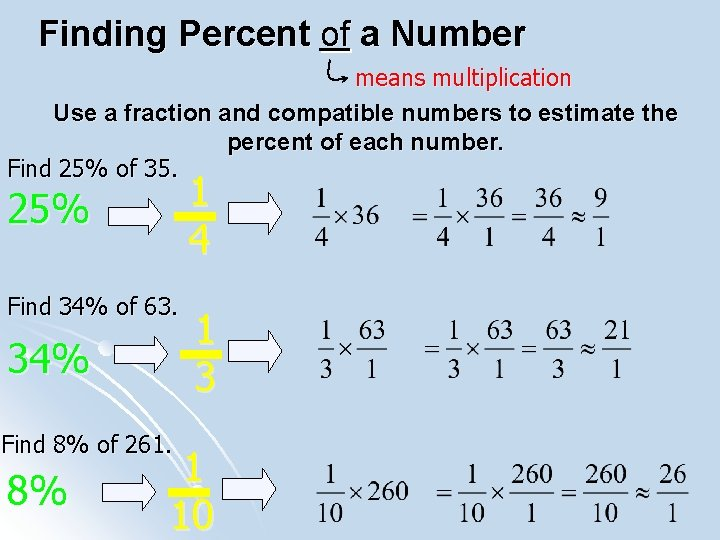# Objective l SWBAT estimate percents Estimating with Percents

• Slides: 8Objective l SWBAT estimate percents“Estimating with Percents” l You can use fractions or decimals to estimate with percents.Estimating Percents with Fractions You can estimate the percent of a number by substituting a fraction that is close to a given percent. Percent 10% 25% 33 % 50% 66 % Fraction A basketball costs \$14. 99 at Modell’s. Sports Authority is offering the same basketball at 20% off the regular price of \$19. 99. Estimate to determine which store is offering the better deal? Find 20% of \$19. 99 Compatible numbers – numbers that are close and help you use mental math to find the solutionFinding Percent of a Number means multiplication Use a fraction and compatible numbers to estimate the percent of each number. Find 25% of 35. 1 4 25% Find 34% of 63. 34% Find 8% of 261. 8% 1 3 1 10Estimating Percents with Decimals Another way to estimate percents is to find 1% or 10% of a number. You can do this by moving the decimal point in the number. 1% Find of any number by moving the decimal point 2 places to the left. Find 1% of 45 45. 0 = 0. 45 10% Find of any number by moving the decimal point 1 place to the left. Find 10% of 45 45. 0 = 4. 5Use 1% or 10% to estimate the percent of each number. Find 4% of 18 18 is about 20, so find 4% of 20. 1% of 20 =. 20. 4% of 20 = 4 · 0. 2 = 0. 8 4% equals 4 · 1%. 4% of 18 is about 0. 8. Find 29% of 80 29% is about 30, so find 30% of 80. 10% of 80 = 80. . 30% of 80 = 3 · 8. 0 = 24. 0 30% equals 3 · 10% 29% of 80 is about 24.Real-Life Application Tim spent \$58 on dinner for his family. About how much money should he leave for a 15% tip? Since \$58 is about \$60, find 15% of \$60. 15% = 10% + 5% 10% of \$60 = \$6 Think: 15% is 10% + 5%. 1 2 5% is of 10% so divide 5% of \$60 = \$6 ÷ 2 = \$3 \$6 by 2. \$6 + \$3 = \$9 Add the 10% and 5% estimates. Tim should leave about \$9 for a 15% tip.Real-Life Application Amanda spent \$12 on a hair cut. About how much money should she leave for a 15% tip? Since \$12 is about \$10, find 15% of \$10. 15% = 10% + 5% 10% of \$10 = \$1 Think: 15% is 10% + 5%. 1 2 5% is of 10% so divide 5% of \$10 = \$1 ÷ 2 = \$0. 50 \$1 by 2. \$1 + \$0. 50 = \$1. 50 Add the 10% and 5% estimates. Amanda should leave about \$1. 50 for a 15% tip.